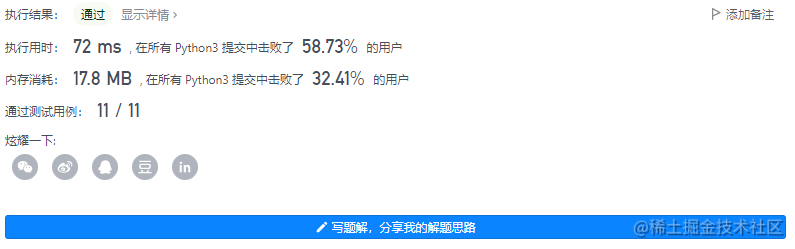# leetcode_346 資料流中的移動平均值

### 要求

MovingAverage(int size) 用視窗大小 size 初始化物件。\ double next(int val) 計算並返回資料流中最後 size 個值的移動平均值。

• 1 <= size <= 1000
• -105 <= val <= 105
• 最多呼叫 next 方法 104 次

### 核心程式碼

```python class MovingAverage: def init(self, size: int): self.queue = deque() self.Max_size = size

``````def next(self, val: int) -> float:
self.queue.append(val)
if len(self.queue) > self.Max_size:
self.queue.popleft()
return 1.0 * sum(self.queue) / len(self.queue)
``````

# param_1 = obj.next(val)

```